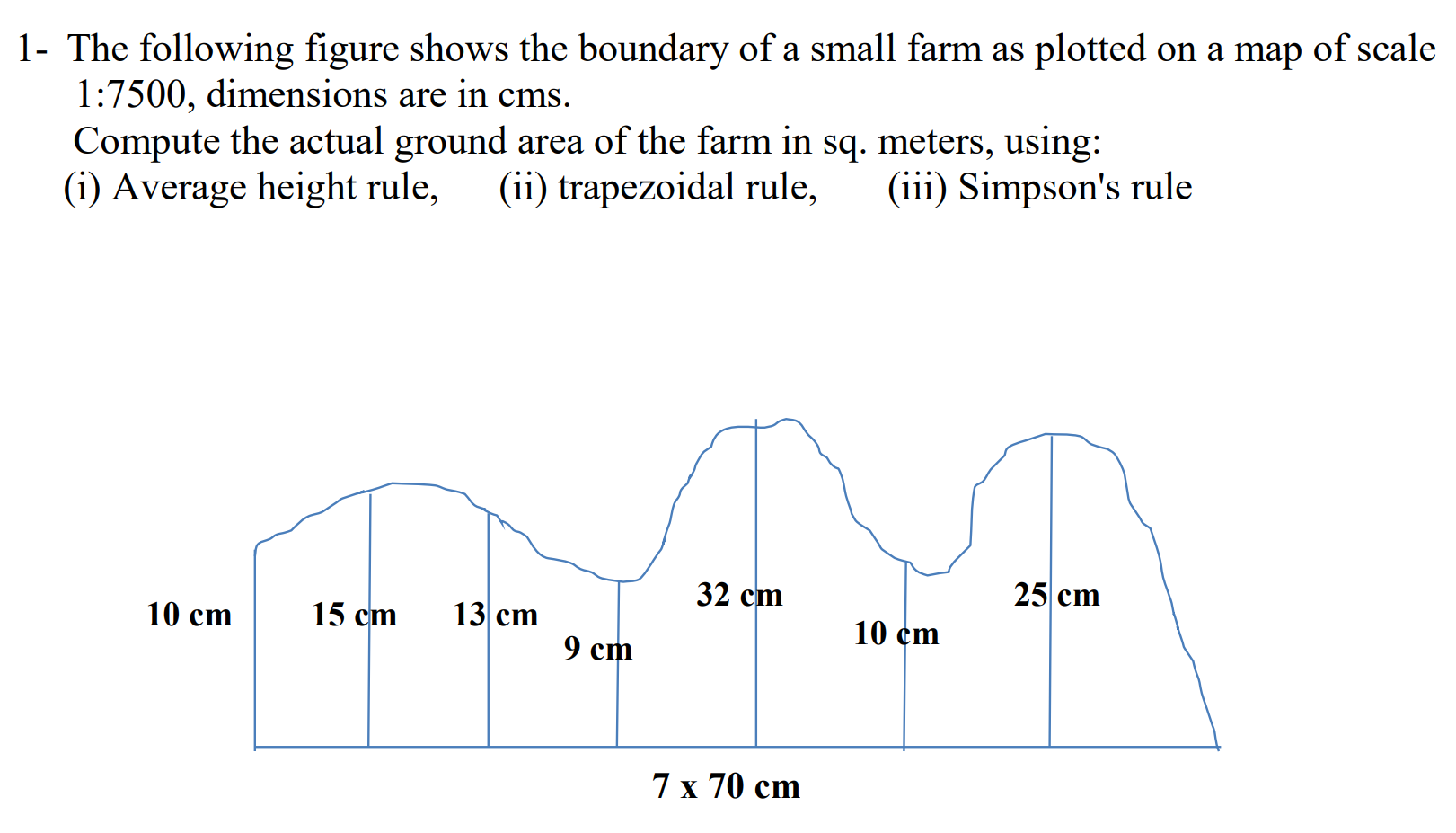Home / Expert Answers / Civil Engineering / 1-the-following-figure-shows-the-boundary-of-a-small-farm-as-plotted-on-a-map-of-scale-1-7500-pa454

# (Solved): 1- The following figure shows the boundary of a small farm as plotted on a map of scale \( 1: 7500 ...1- The following figure shows the boundary of a small farm as plotted on a map of scale \( 1: 7500 \), dimensions are in cms. Compute the actual ground area of the farm in sq. meters, using: (i) Average height rule, (ii) trapezoidal rule, (iii) Simpson's rule

We have an Answer from Expert